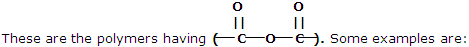electrochemistry ( electrolysis of metals)

1. Define Faraday's first law of electrolysis 2. define Faraday's second law of electrolysis

#### Related Questions in Chemistry

• ##### Q :Unit of molality Select the right

Select the right answer of the question. The unit of molality is: (a) Mole per litre (b) Mole per kilogram (c) Per mole per litre (d) Mole litre

• ##### Q :Lowering of vapour pressure Help me to

Help me to go through this problem. Lowering of vapour pressure is highest for: (a) urea (b) 0.1 M glucose (c) 0.1M MgSo4 (d) 0.1M BaCl2

• ##### Q :Ionic radius of chloride ion The edge

The edge length of the unit cell of Nacl crystal lattice is 552 pm. If ionic radius of sodium ion is 95. What is the ionic radius of chloride ion:(a) 190 pm  (b) 368 pm  (c) 181 pm  (d) 276 pm     <

• ##### Q :What are condensation polymers? Give

These types of polymers are formed as a result of condensation reaction between monomer units. Some common examples are being discussed here: 1. Polyesters##### Q :State octet rule in chemistry Explain

Explain what is octet rule in chemistry?

• ##### Q :Explain the process of adsorption of

The extent of adsorption of a gas on a solid adsorbent is affected by the following factors: 1. Nature of the gas Since physical adsorption is non-specific in nature, every gas will get adsorbed on the

• ##### Q :Q what is the basicity of primary

what is the basicity of primary secondary and tertiary amines in chlorobenzene

• ##### Q :Relative lowering in vapour pressure of

Give me answer of this question. "Relative lowering in vapour pressure of solution containing non-volatile solute is directly proportional to mole fraction of solute". Above statement is: (a) Henry law (b) Dulong and Petit law (c) Raoult's law (d) Le-Chatelier's pri

• ##### Q :Explain Photoelectron Spectroscopy. The

The energies of both the outer and inner orbitals of atoms and molecules can be determined by photoelectron spectroscopy.Energy changes of the outermost or highest energy electron of molecules were dealt with here in a different passion. The energies of ot

• ##### Q :Molecular Symmetry Types The number of

The number of molecular orbitals and molecular motions of each symmetry type can be deduced. Let us continue to use the C2v point group and the H2O molecule to illustrate how the procedure develop